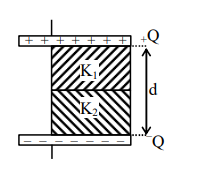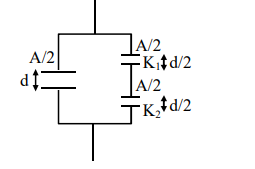# A parallel - plate capacitor with plate area A has separation

Question:

A parallel - plate capacitor with plate area A has separation $\mathrm{d}$ between the plates. Two dielectric slabs of dielectric constant $K_{1}$ and $K_{2}$ of same area $\mathrm{A} / 2$ and thickness $\mathrm{d} / 2$ are inserted in the space between the plates. The capacitance of the capacitor will be given by :1. $\frac{\varepsilon_{0} \mathrm{~A}}{\mathrm{~d}}\left(\frac{1}{2}+\frac{\mathrm{K}_{1} \mathrm{~K}_{2}}{\mathrm{~K}_{1}+\mathrm{K}_{2}}\right)$

2. $\frac{\varepsilon_{0} \mathrm{~A}}{\mathrm{~d}}\left(\frac{1}{2}+\frac{\mathrm{K}_{1} \mathrm{~K}_{2}}{2\left(\mathrm{~K}_{1}+\mathrm{K}_{2}\right)}\right)$

3. $\frac{\varepsilon_{0} \mathrm{~A}}{\mathrm{~d}}\left(\frac{1}{2}+\frac{\mathrm{K}_{1}+\mathrm{K}_{2}}{\mathrm{~K}_{1} \mathrm{~K}_{2}}\right)$

4. $\frac{\varepsilon_{0} \mathrm{~A}}{\mathrm{~d}}\left(\frac{1}{2}+\frac{2\left(\mathrm{~K}_{1}+\mathrm{K}_{2}\right)}{\mathrm{K}_{1} \mathrm{~K}_{2}}\right)$

Correct Option: 1

Solution:

$\mathrm{C}_{\mathrm{eq}}=\frac{\frac{\mathrm{A}}{2} \varepsilon_{0}}{\mathrm{~d}}+\frac{\mathrm{A} \varepsilon_{0}}{\mathrm{~d}} \frac{\mathrm{K}_{1} \mathrm{~K}_{2}}{\mathrm{~K}_{1}+\mathrm{K}_{2}}$

$=\frac{\mathrm{A} \varepsilon_{0}}{\mathrm{~d}}\left(\frac{1}{2}+\frac{\mathrm{K}_{1} \mathrm{~K}_{2}}{\mathrm{~K}_{1}+\mathrm{K}_{2}}\right)$Ans.1